Minors and Cofactors of Determinants

• Last Updated : 21 Feb, 2021

Matrix is an array of real numbers(or other suitable entities), arranged in rows and columns where entities are referred to elements present in the Matrix. Below image demonstrates the Matrix, where elements separated horizontally are known as rows of the Matrix and the elements separated vertically are know as columns of the Matrix.

As we know Matrix is arranged in rows and columns, the below matrix having 3 rows and 3 columns, So the order of the Matrix is 3 × 3.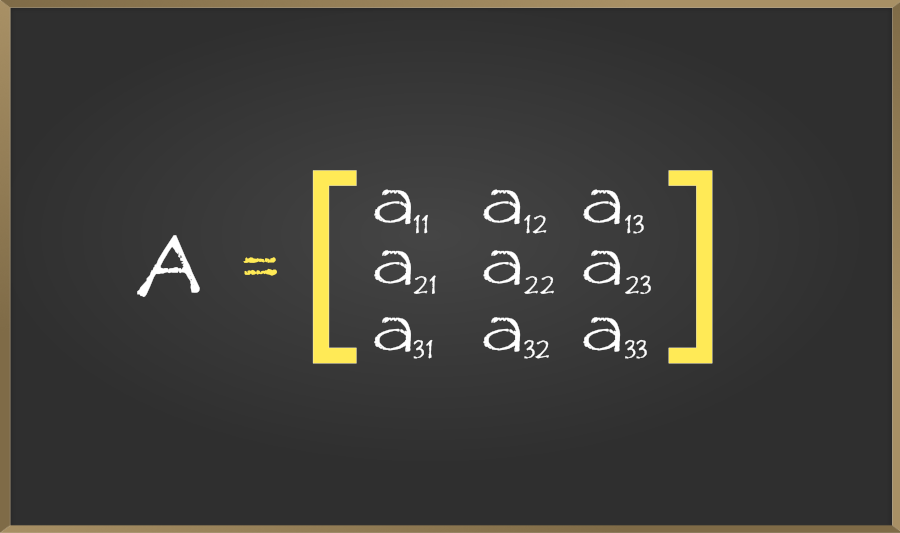Any four elements a, b, c, and d are arranged in two rows and two columns between two Vertical bars as shown below, forms which are called Determinant of the second-order or second-order determinant. As shown below demonstrating the Determinant and expansion of Determinant.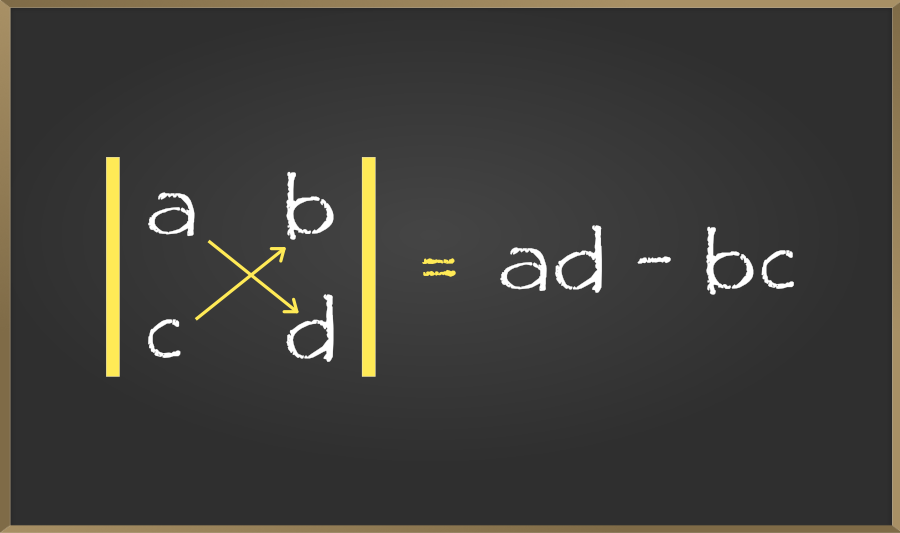Determinant of a Matrix

Determinant is useful for solving linear equations, capturing how linear transformation change area or volume, and changing variables in integrals. The determinant can be viewed as a function whose input is a square matrix and whose output is a number. In the below article we are discussing the Minors and Cofactors thoroughly. In simple language we can say, To every small matrix A, we can associate a number (real or complex) which is called the determinant of a square matrix A.

Determinant of a matrix can be easily represented as det (A) or | A |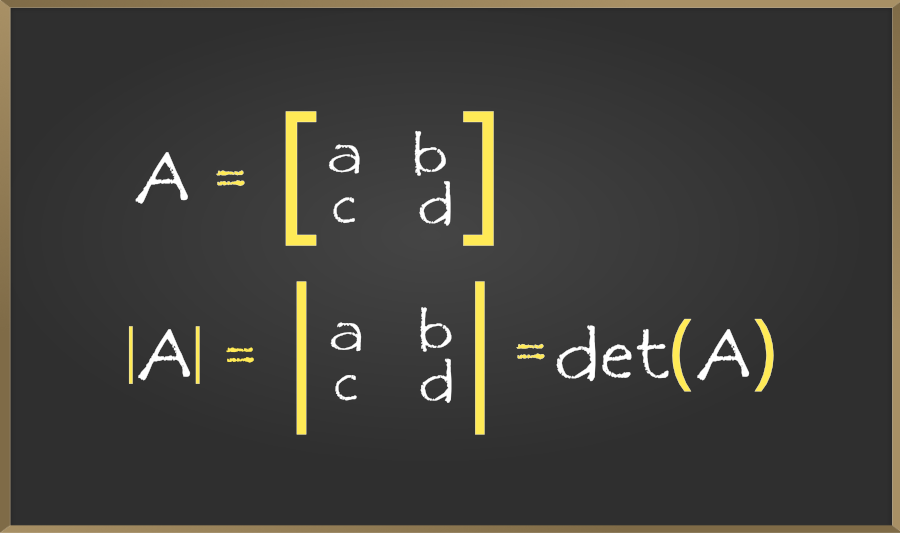So firstly let’s discuss the Minors.

Note:

• The questions which are done in this article have appeared in different previous year question papers.
• i represent rows of the determinant whereas j represents columns of the determinant.
• I am highlighting the ijth terms so that you can see clearly without any confusion.
• In the below article you will see the questions and solution of that question is demonstrated by the image.

Minor of a Matrix

Minor of an element aij of a determinant, is a determinant obtained by deleting the ith row and jth column in which element aij lies. Minor of an element aij is denoted by Mij

Steps for Computing Minor of a Matrix

Step 1: Hide the ith row and jth column of the matrix A, where the element aij lies.

Step 2: Now compute the determinant of the matrix after the row and column is removed using step 1.

Sample Problems on Minor of a Matrix

Problem 1: If the matrix A is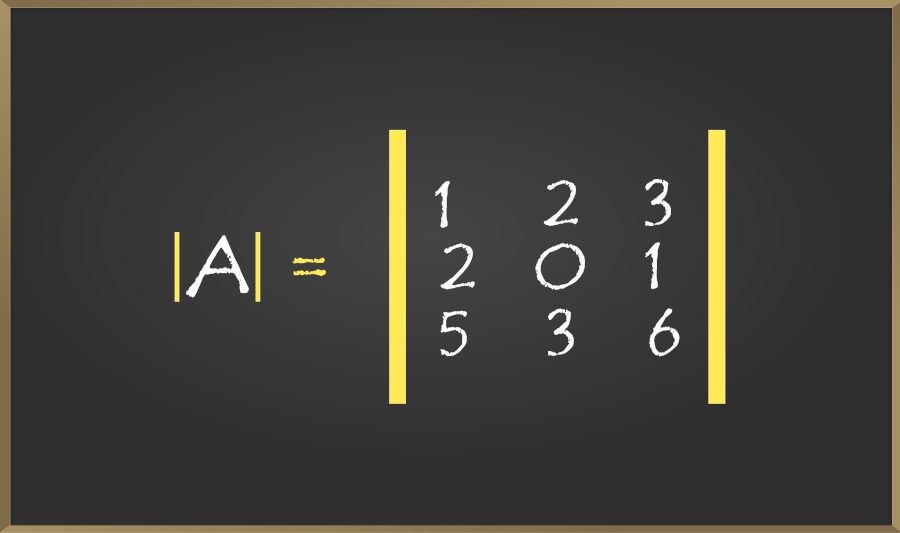then, write the minor of a22.

Solution:

In this question, we have to find out the minor of a22, the element present at a22 is 0. As we learn from our definition of a minor we have to delete the ith row and jth columns at which our asked element is present. Below image is demonstrating how to delete the ith row and jth column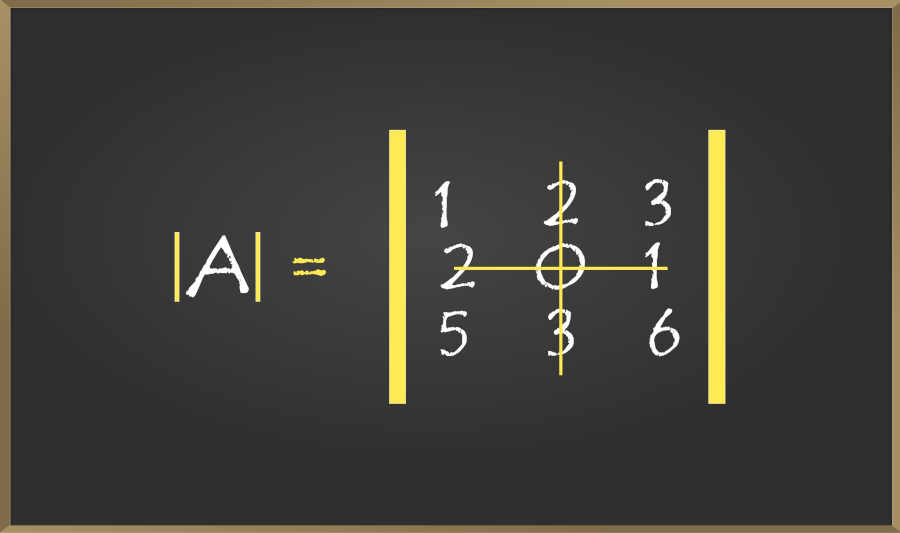After deletion, we write our left element as it is and do cross multiplication.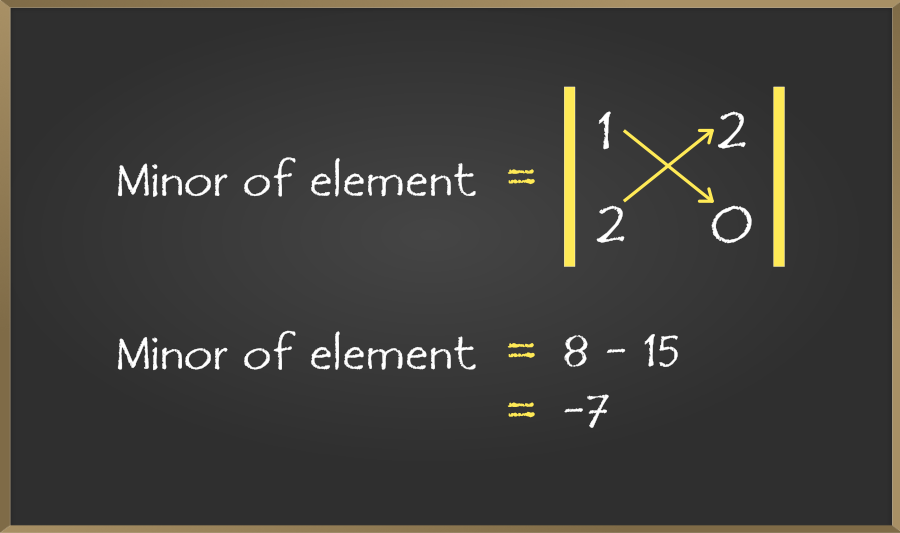Now after deleting ith row and jth column we had to expand the determinant, so we get (8 – 15) which on solving gives -7, which is our required answer.

Note: Always remember, after multiplication of left diagonal element always put -ve sign then do the multiplication of right diagonal elements and solve them out.

Problem 2: If the matrix A is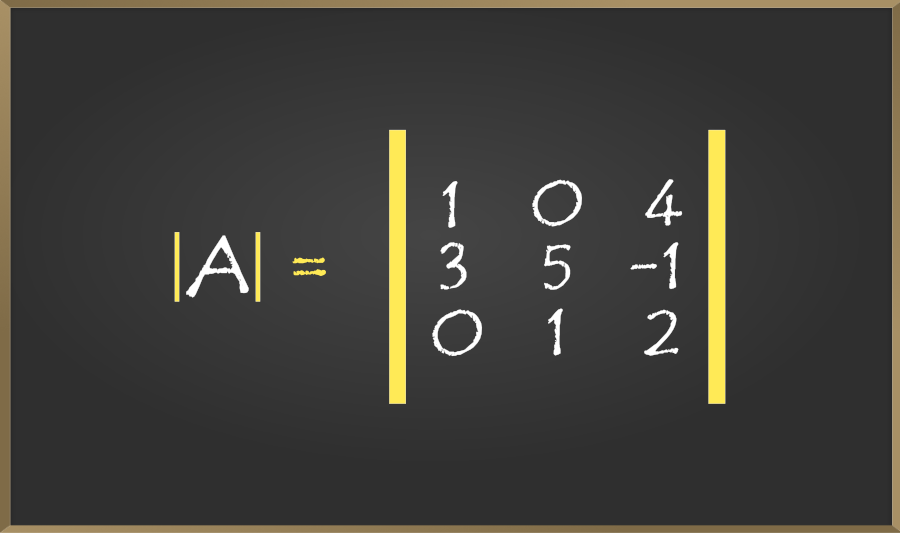then find out minor of a32.

Solution:

In the above question we have asked to find out the minor of a32 element which is 1. So as we did in this above problem same procedure we will follow. So firstly we have to delete the ith row and jth column at which our element is present.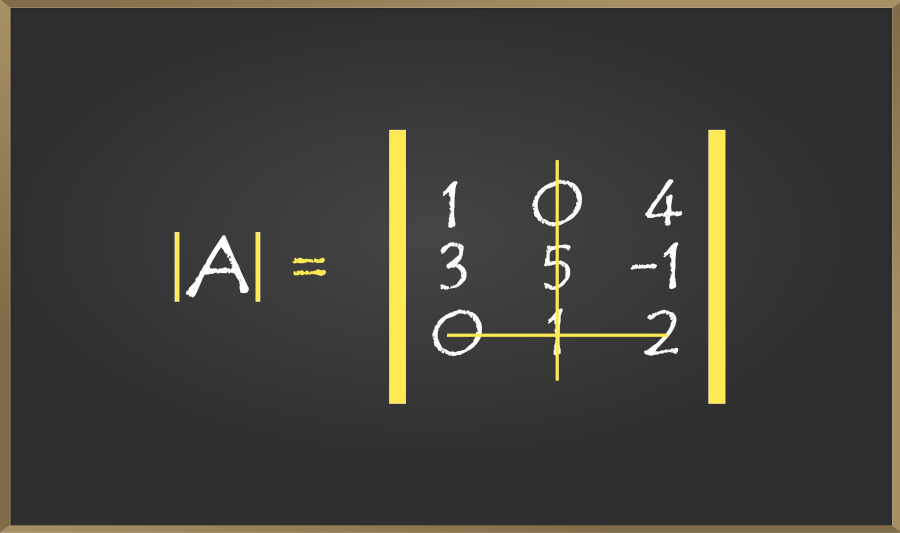So we had canceled the ith row and jth column at which our element is present. So write the elements which are left as it is.

Then do the cross multiplication and solve: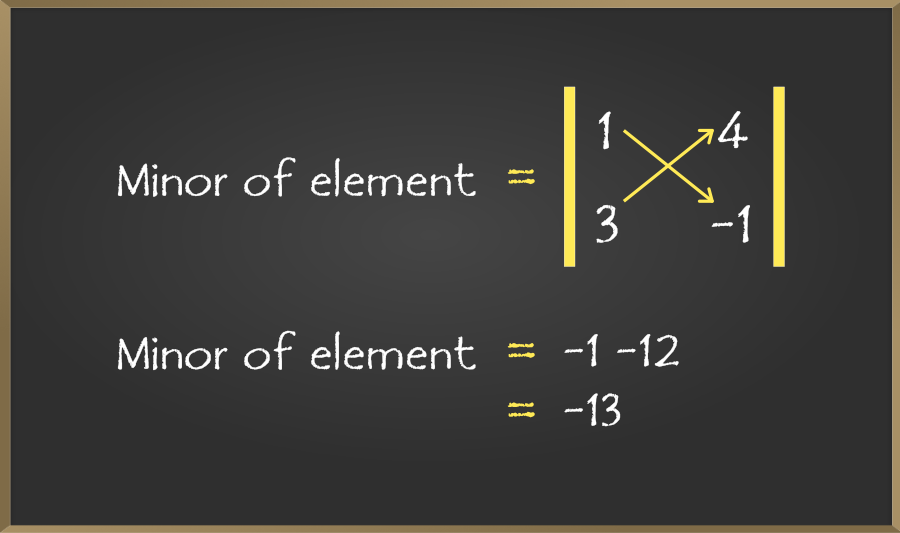By following the same procedure as of the above question we had solved this question too through the expansion of determinant as we discussed in our Introduction.

Co-factors of a Matrix

Co-factor of an element aij of a determinant, denoted by Aij or Cij , is defined as Aij = (-1)i+j Mij , where Mij is a minor of an element aij

Formula to find cofactors

Aij = (-1)i+j  Mij

Sample Problems on Co-factors of a Matrix

Problem 1: If a matrix A is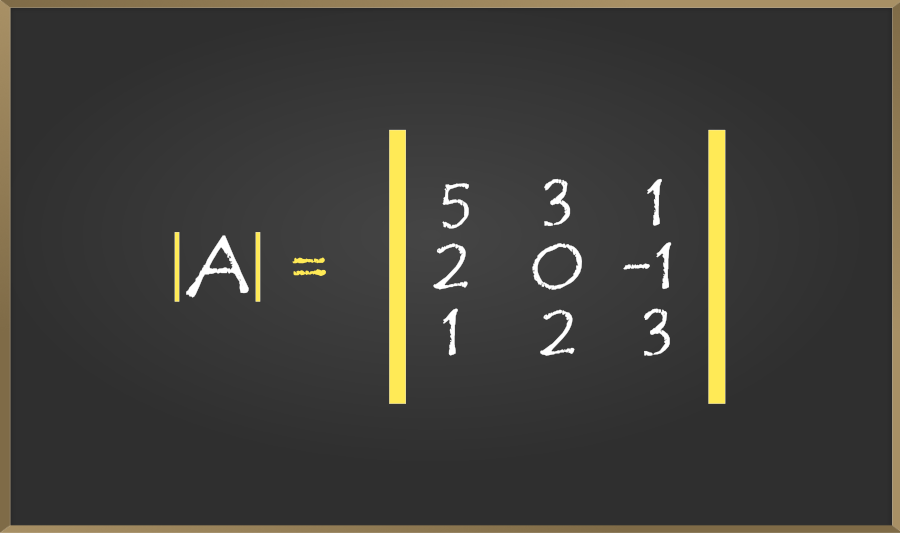write the cofactor of the element a32.

Solution:

As asked in question we have to find the co factor of element a32 which means our row (i) = 3 and column (j) = 2 so we have row and column as we do to find the minor by deleting the rows and column at which asked element exist we do the same in this question to and then put that in our formula -> Aij = (-1)i+j  Mij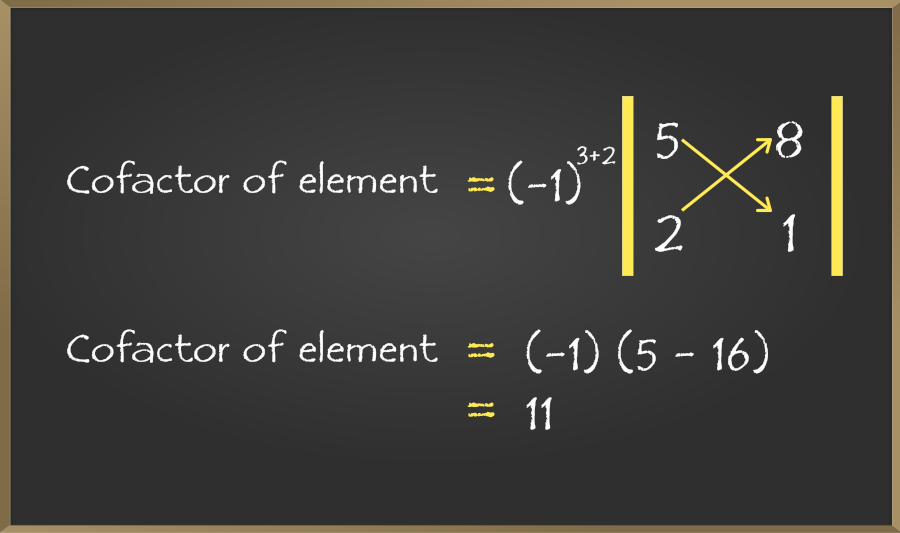So after putting in the formula of finding cofactor and doing expansion of determinant we get (-1) (5 – 16) which on solving gives the answer 11, this is our required answer.

Problem 2: If Aij of the element aij of the determinant given below, then write the value of a32 . A32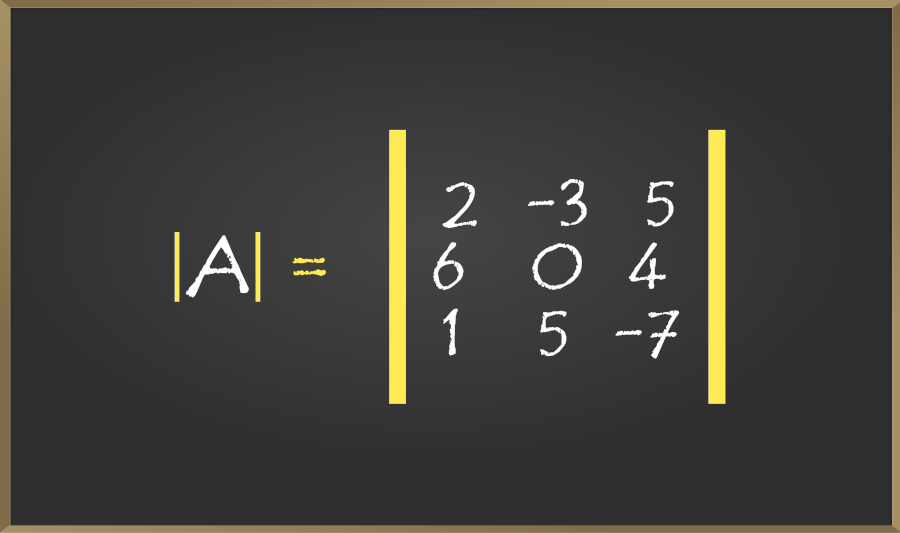Solution:

In the question, we are having determinant. So we have row and column given in the question.

Here, a32 = 3+2 = 5

Given, Aij is the cofactor of the element aij of A . So now we can solve this question by putting the values in the formula of cofactor as discussed in above question.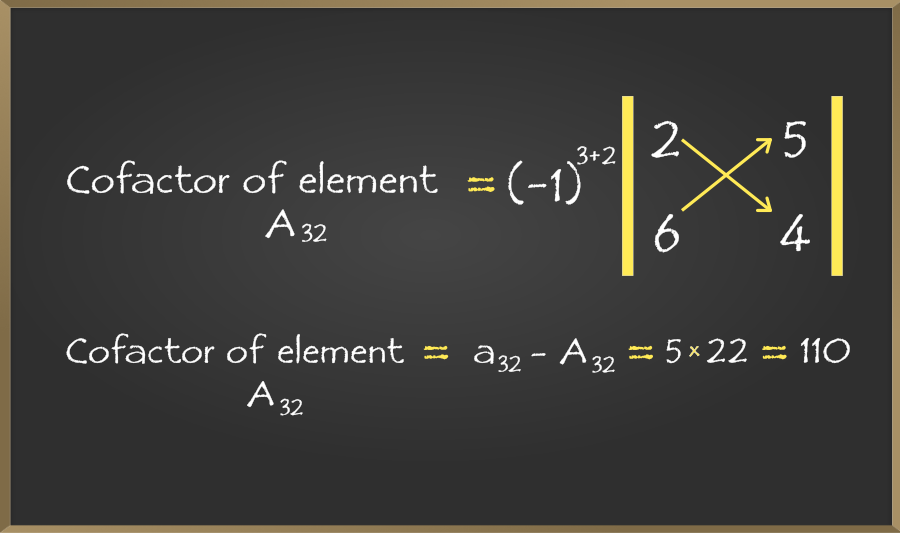So, 110 is our required answer.

My Personal Notes arrow_drop_up